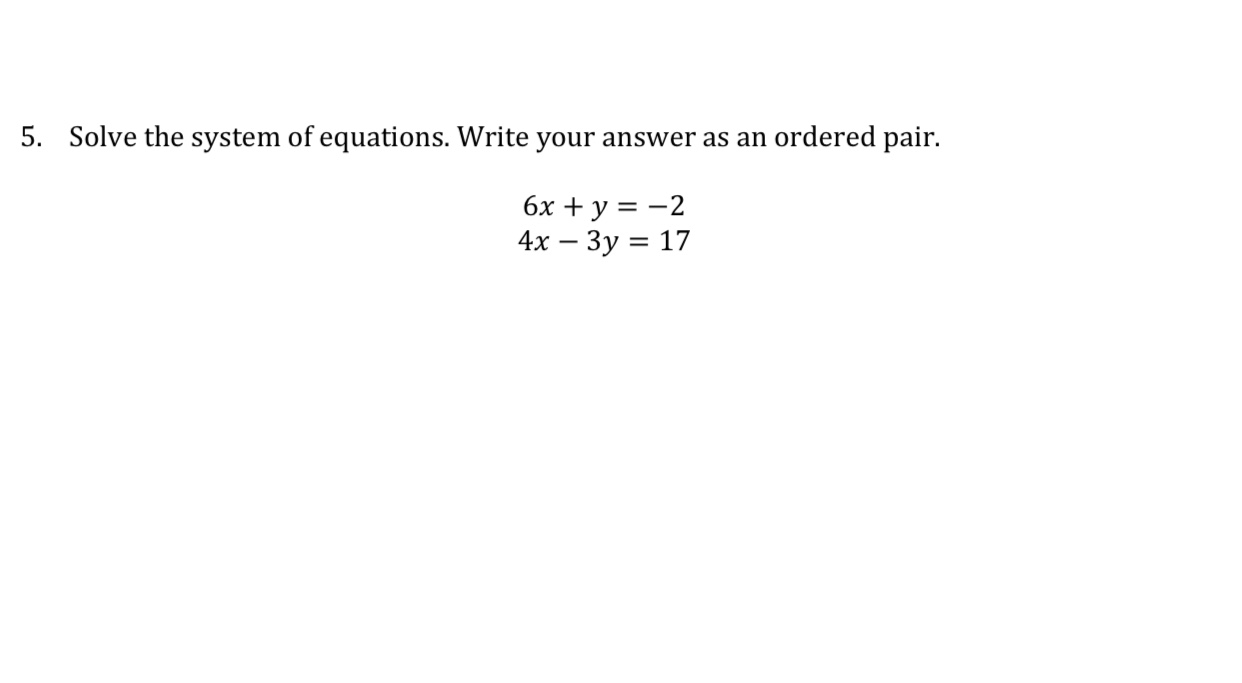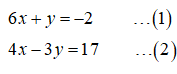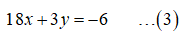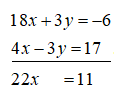# 5.Solve the system of equations. Write your answer as an ordered pair.6x + y = -24х — Зу 3D 17

Question
18 viewshelp_outlineImage Transcriptionclose5. Solve the system of equations. Write your answer as an ordered pair. 6x + y = -2 4х — Зу 3D 17 fullscreen
check_circle

Step 1
1. The given system of equations is,Step 2

Multiply the equation (1) by 3.Step 3

Add the two equations (3) and (2)....

### Want to see the full answer?

See Solution

#### Want to see this answer and more?

Solutions are written by subject experts who are available 24/7. Questions are typically answered within 1 hour.*

See Solution
*Response times may vary by subject and question.
Tagged in

### Algebra